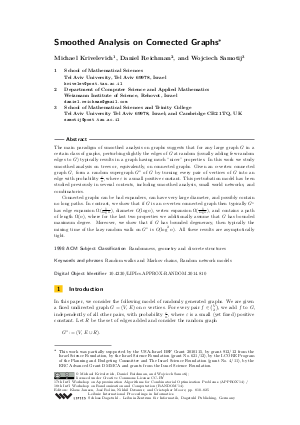Document# Smoothed Analysis on Connected Graphs

### Authors Michael Krivelevich, Daniel Reichman, Wojciech Samotij## File

LIPIcs.APPROX-RANDOM.2014.810.pdf
• Filesize: 491 kB
• 16 pages

## Cite As

Michael Krivelevich, Daniel Reichman, and Wojciech Samotij. Smoothed Analysis on Connected Graphs. In Approximation, Randomization, and Combinatorial Optimization. Algorithms and Techniques (APPROX/RANDOM 2014). Leibniz International Proceedings in Informatics (LIPIcs), Volume 28, pp. 810-825, Schloss Dagstuhl - Leibniz-Zentrum für Informatik (2014)
https://doi.org/10.4230/LIPIcs.APPROX-RANDOM.2014.810

## Abstract

The main paradigm of smoothed analysis on graphs suggests that for any large graph G in a certain class of graphs, perturbing slightly the edges of G at random (usually adding few random edges to G) typically results in a graph having much "nicer" properties. In this work we study smoothed analysis on trees or, equivalently, on connected graphs. Given an n-vertex connected graph G, form a random supergraph of G* of G by turning every pair of vertices of G into an edge with probability epsilon/n, where epsilon is a small positive constant. This perturbation model has been studied previously in several contexts, including smoothed analysis, small world networks, and combinatorics. Connected graphs can be bad expanders, can have very large diameter, and possibly contain no long paths. In contrast, we show that if G is an n-vertex connected graph then typically G* has edge expansion Omega(1/(log n)), diameter O(log n), vertex expansion Omega(1/(log n)), and contains a path of length Omega(n), where for the last two properties we additionally assume that G has bounded maximum degree. Moreover, we show that if G has bounded degeneracy, then typically the mixing time of the lazy random walk on G* is O(log^2(n)). All these results are asymptotically tight.
##### Keywords
• Random walks and Markov chains
• Random network models

## Metrics

• Access Statistics
• Total Accesses (updated on a weekly basis)
0